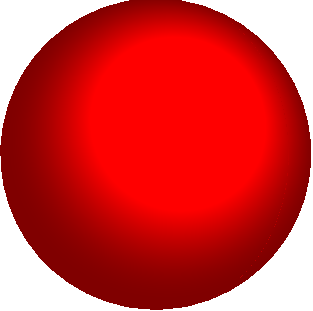Chapter 3

Constitutive Models $–$ Relations between Stress and Strain

3.4 Generalized Hooke’s law $–$  elastic materials subjected to small stretches but large rotations

Recall that the stress-strain law for an anisotropic, linear elastic material (Sect 3.1) has the form

${\sigma }_{ij}={C}_{ijkl}{\epsilon }_{kl}$

where ${\sigma }_{ij}$ is stress (any stress measure you like), ${\epsilon }_{ij}=\left(\partial {u}_{i}/\partial {x}_{j}+\partial {u}_{j}/\partial {x}_{i}\right)/2$ is the infinitesimal strain, and ${C}_{ijkl}$ is the tensor of elastic moduli.This stress-strain relation can only be used if the material is subjected to small deformations, and small rotations.  This is partly because the infinitesimal strain ${\epsilon }_{ij}\ne 0$ for a finite rotation $–$ so the law predicts that a nonzero stress is required to rotate a solid.

There are some situations where a solid is subjected to small shape changes, but large rotations.  For example, the picture shows a long slender beam bent into a circle by moments applied to its ends.  The strains in the beam are of order $h/R$, where h is the thickness of the beam and R is its curvature.   The ends of the beam have rotated through a full 90 degrees, however.   The linear elastic constitutive equations would not predict the correct stress in the beam.It is easy to fix this problem: provided we choose a sensible (nonlinear) strain measure, together with the appropriate work-conjugate stress measure, we can still use a linear stress-strain relation.  To make this precise, suppose that a solid is subjected to a displacement field ${u}_{i}\left({x}_{k}\right)$.  DefineThe deformation gradient and its jacobian

${F}_{ij}={\delta }_{ij}+\frac{\partial {u}_{i}}{\partial {x}_{j}}$       $J=\mathrm{det}\left(F\right)$The Lagrange strain

${E}_{ij}=\frac{1}{2}\left({F}_{ki}{F}_{kj}-{\delta }_{ij}\right)\text{\hspace{0.17em}}\text{\hspace{0.17em}}=\frac{1}{2}\left(\frac{\partial {u}_{i}}{\partial {x}_{j}}+\frac{\partial {u}_{j}}{\partial {x}_{i}}+\frac{\partial {u}_{k}}{\partial {x}_{i}}\frac{\partial {u}_{k}}{\partial {x}_{j}}\right)$The Eulerian strain $\text{\hspace{0.17em}}\text{\hspace{0.17em}}\text{\hspace{0.17em}}{E}_{ij}^{*}=\frac{1}{2}\left({\delta }_{ij}-{F}_{ki}^{-1}{F}_{kj}^{-1}\right)$The rotation tensor (see 2.1.13 for the best way to compute R in practice)

${R}_{ij}={F}_{ik}{U}_{kj}^{-1}\text{\hspace{0.17em}}\text{\hspace{0.17em}}\text{\hspace{0.17em}}\text{\hspace{0.17em}}\text{\hspace{0.17em}}\text{\hspace{0.17em}}\text{\hspace{0.17em}}\text{\hspace{0.17em}}\text{\hspace{0.17em}}\text{\hspace{0.17em}}U={\left({F}^{T}F\right)}^{1/2}$The Cauchy (“true”) stress ${\sigma }_{ij}$, defined so that  ${n}_{i}{\sigma }_{ij}=\underset{dA\to 0}{Lim}\frac{d{P}_{j}^{\left(n\right)}}{dA}$The Material stress (work conjugate to Lagrange strain)  ${\Sigma }_{ij}=J{F}_{ik}^{-1}{\sigma }_{kl}{F}_{jl}^{-1}$

The Material stress-Lagrange strain relation can be expressed as

${\Sigma }_{ij}={C}_{ijkl}{E}_{kl}$

where ${C}_{ijkl}$ is the tensor of elastic moduli for the material with orientation in the undeformed configuration.  This is identical to the stress-strain relation for a linear elastic solid, except that the stress measure has been replaced by Material stress, and the strain measure has been replaced by Lagrange strain. You can therefore use all the matrix representations and tables of data given in Section 3.1 to apply the constitutive equation. The Cauchy (“true”) stress can be computed from the material stress as

${\sigma }_{ij}=\frac{1}{J}{F}_{ik}{\Sigma }_{kl}{F}_{jl}\approx {R}_{ik}{\Sigma }_{kl}{R}_{jl}$

The Cauchy stress-Eulerian strain relation: Alternatively, the stress-strain relation can be expressed in terms of stress and deformation measures that characterize the deformed solid, as

${\sigma }_{ij}={C}_{ijkl}^{*}{E}_{kl}^{*}$

where ${C}_{ijkl}^{*}$ is the tensor of elastic moduli for the material with orientation of the deformed configuration.  This tensor is related to ${C}_{ijkl}$ by

${C}_{ijmn}^{*}=\frac{1}{J}{F}_{ip}{F}_{jq}{C}_{pqkl}{F}_{mk}{F}_{nl}\approx {R}_{ip}{R}_{jq}{C}_{pqkl}{R}_{mk}{R}_{nl}$

For the special case of an isotropic material with Young’s modulus E and Poisson’s ratio $\nu$

${\Sigma }_{ij}=\frac{E}{1+\nu }\left\{{E}_{ij}+\frac{\nu }{1-2\nu }{E}_{kk}{\delta }_{ij}\right\}\text{\hspace{0.17em}}\text{\hspace{0.17em}}\text{\hspace{0.17em}}\text{\hspace{0.17em}}\text{\hspace{0.17em}}\text{\hspace{0.17em}}\text{\hspace{0.17em}}\text{\hspace{0.17em}}\text{\hspace{0.17em}}\text{\hspace{0.17em}}\text{\hspace{0.17em}}\text{\hspace{0.17em}}\text{\hspace{0.17em}}\text{\hspace{0.17em}}\text{\hspace{0.17em}}\text{\hspace{0.17em}}{\sigma }_{ij}=\frac{E}{1+\nu }\left\{{E}_{ij}^{*}+\frac{\nu }{1-2\nu }{E}_{kk}^{*}{\delta }_{ij}\right\}$